Redox reactions 2010 VCE 1) In a problem-solving activity a student is given the following information regarding three half-equations. However, although the three numerical values of E0 are correct, they have been incorrectly assigned to the three half-equations.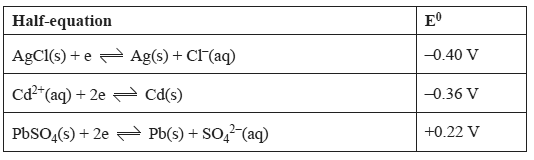The objective of this task is to correctly assign the E0 values to the corresponding half-equation. To do this, the student constructs standard half-cells for each of the above half-reactions. These half-cells are connected, one at a time, to a standard hydrogen half-cell as indicated in the diagram below.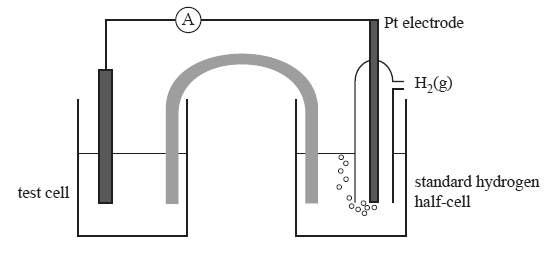The following observations were made either during or after the electrochemical cell discharged electricity for several minutes.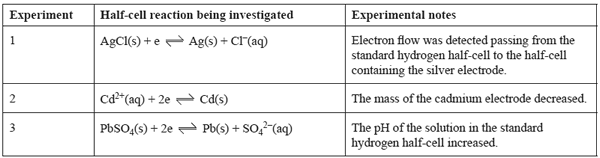a. The above information can only be used to assign one of the E0 values to its corresponding half-equation. Identify this half-equation by placing the correct E0 value next to its corresponding half-equation in the table below.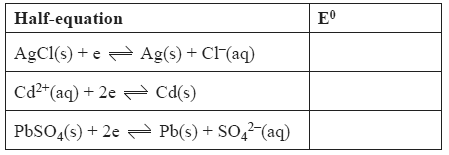Solution b) Explain why the other two E0 values cannot be correctly assigned to their half-equations. Solution c) Explain why the pH of the solution in the standard hydrogen half-cell increased in experiment 3. Solution2) The lithium button cell, used to power watches and calculators, is a primary cell containing lithium metal. The lithium ion cell is a secondary cell that is used to power laptop computers. a. What is the difference between a primary and secondary cell? Solution b. By referring to information provided in the Data Book, give one reason why lithium is used as a reactant in these galvanic cells. Solution Some early lithium metal batteries exploded when exposed to water. c. Write a balanced equation, including states, for the reaction between lithium metal and water to explain why anexplosion might occur. SolutionIn lithium ion cells, lithium ions move between the electrodes as the cell is discharged and recharged. The negative electrode consists of lithiated graphite, LiC6 , and the positive electrode consists of lithium cobalt oxide, LiCoO2. The chemical reactions that take place in the lithium ion cell are complex. The following equations present a simplified description of the reactions that occur at the electrodes as the cell is recharged. positive electrode LiCoO2 → CoO2+ Li+ + e– negative electrode 6C + Li+ + e– → LiC6 d. On the diagram below, use arrows to indicate the directions of movement of electrons, e–, and Li+ ions as the lithium ion cell is discharged.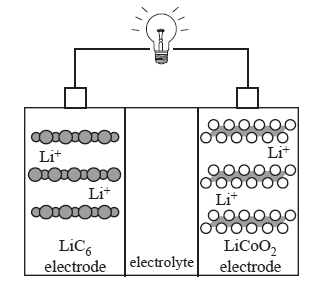Solution Lithium metal is produced by the electrolysis of molten lithium chloride, LiCl. e. Calculate the mass of lithium metal produced in 48.0 hours using a current of 6.50 amps. Solution